# Short Answer Type Questions: Quadrilaterals - Notes | Study Mathematics (Maths) Class 9 - Class 9

 1 Crore+ students have signed up on EduRev. Have you?

Question 1. In the adjoining figure, if ∠ B = 68°, then find ∠ A, ∠ C and ∠ D.
Solution:
∵ Opposite angles of a parallelogram are equal.
∴ ∠ B= ∠ D
⇒ ∠ D = 68°                           [∵ ∠B = 68°,    given]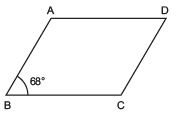∵ ∠ B and ∠ C are supplementary.
∴ ∠ B + ∠ C = 180°
⇒ ∠ C = 180° - ∠ B = 180° - 68° = 112°
Since ∠A and ∠C are opposite angles.
∴ ∠ A= ∠ C
⇒ ∠ A = 112°                                 [∵ ∠ C = 112°]
Thus, ∠ A = 112°, ∠ D = 68° and ∠ C = 112°

Question 2. In the figure, ABCD is a parallelogram. If AB = 4.5 cm, then find other sides of the parallelogram when its perimeter is 21 cm.
Solution:
∵ Opposite sides of a parallelogram are equal.
∴ AB = CD = 4.5 cm, and BC = AD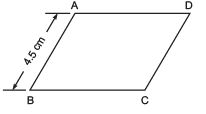Now, AB + CD + BC + AD = 21 cm
⇒ AB + AB + BC + BC = 21 cm
⇒2[AB + BC] = 21 cm
⇒ 2[4.5 cm + BC] = 21 cm
⇒ [4.5 cm + BC] = (21/2)
= 11.5 cm ⇒ BC = 11.5 ∠ 4.5 = 7 cm
Thus, BC = 7 cm, CD = 4.5 cm and AD = 7 cm.

Question 3. In a parallelogram ABCD,if (3x ∠ 10)° = ∠ B and (2x + 10)° = ∠ C, then find the value of x.
Solution:
Since, the adjacent angles of a parallelogram are supplementary.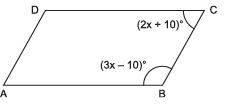∴ ∠ B + ∠ C = 180°
⇒ (3x - 10)° + (2x + 10)° = 180°
⇒ 3x + 2x - 10° + 10° = 180°
⇒ 5x = 180°
⇒ x= (1800/5)= 36°
Thus, the required value of x is 36°.

Question 4. The adjoining figure is a rectangle whose diagonals AC and BD intersect at O. If ∠ OAB = 27°, then find ∠ OBC.
Solution:
Since, the diagonals of a rectangle are equal and bisect each other.
∴ OA = OB
⇒ ∠ OBA = ∠ OAB = 27°
Also, each angle of a rectangle measures 90°.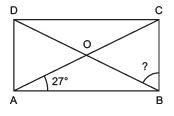∴ ∠ ABC = 90°
⇒ ∠ ABO + ∠ CBO = 90°
⇒ ∠ OBA + ∠ OBC = 90°
⇒ 27° + ∠ OBC = 90°
⇒ ∠ OBC = 90° - 27° = 63°

Question 5. In a quadrilateral, ∠ A : ∠ B : ∠ C : ∠ D = 1 : 2 : 3 : 4, then find the measure of each angle of the quadrilateral.
Solution:
Since ∠ A : ∠ B : ∠ C : ∠ D = 1 : 2 : 3 : 4

∴ If ∠ A = x, then ∠ B = 2x, ∠ C = 3x and ∠ D = 4x. ∴ ∠ A + ∠ B + ∠ C + ∠ D = 360°
⇒ x + 2x + 3x + 4x = 360° ⇒ 10x = 36°
⇒ x= (3600/10)= 36°
∴ ∠ A = x = 36° ∠ B = 2x = 2 x 36° = 72° ∠ C = 3x = 3 x 36° = 108° ∠ D = 4x = 4 x 36° = 144°

Question 6. In the figure, D is the mid-point of AB and DE || BC. Find x and y.
Solution:
Since DE || BC and D is the mid-point of AB.
∴ E must be the mid-point of AC.
∴ AE = EC ⇒ x = 5 cm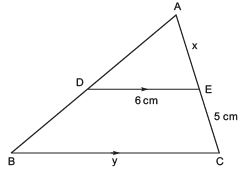Also, DE || BC ⇒ DE = (1/2)BC
∴ 2DE = 2(((1/2))BC)
⇒ 2DE = BC
⇒ 2 x 6 cm = BC or BC = 12 cm
⇒ y = 12 cm
Thus, x = 5 cm and y = 12 cm

Question 1. In the adjoining figure, ABCD is a trapezium in which AB || CD. If ∠ A = 36° and ∠ B = 81°, then find ∠ C and ∠ D.
Solution:
∵ AB || CD and AD is a transversal.            [∵ ABCD is a trapezium in which AB || CD]
∴ ∠ A + ∠ D = 180°
⇒ ∠ D = 180° -  ∠ A = 180° - 36° = 144°
Again, AB || CD and BC is a transversal.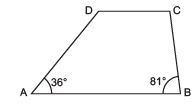∴ ∠ B + ∠ C = 180°
⇒ ∠ C = 180° - ∠ B = 180° - 81° = 99°
∴ The required measures of ∠ D and ∠ C are 144° and 99° respectively.

Question 2. In the figure, the perimeter of D ABC is 27 cm. If D is the mid-point of AB and DE || BC, then find the length of DE.
Solution:
Since, D is the mid-point of AB and DE || BC.
∴ E is the mid-point of AC, and DE = (1/2) BC.
Since, perimeter of DABC = 27 cm
∴ AB + BC + CA = 27 cm
⇒ 2(AD) + BC + 2(AE) = 27 cm
⇒ 2(4.5 cm) + BC + 2(4 cm) = 27 cm
⇒ 9 cm + BC + 8 cm = 27 cm
∴ BC = 27 cm - 9 cm - 8 cm = 10 cm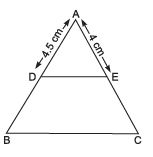∴  (1/2)BC =(10/2) = 5 cm
⇒ DE = 5 cm

Question 3. In the adjoining figure, DE || BC and D is the mid-point of AB. Find the perimeter of ΔABC when AE = 4.5 cm.
Solution:
∵ D is the mid-point of AB and DE || BC.
∴ E is the mid-point of AC and DE = (1/2)BC.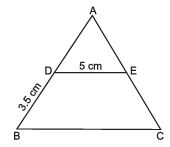⇒ 2DE = BC
⇒ 2 x 5 cm = BC
⇒ BC = 10 cm
Now DB = 3.5 cm
∴ AB = 2(DB) = 2 x 3.5 cm = 7 cm            [D is the mid-point of AB]
Similarly, AC = 2(AE) = 2 x 4.5 cm = 9 cm
Now, perimeter of ΔABC = AB + BC + CA = 7 cm + 10 cm + 9 cm = 26 cm

Question 4. If an angle of a parallelogram is (4/5) of its adjacent angle, then find the measures of all the angles of the parallelogram.
Solution:
Let ABCD is a parallelogram in which ∠ B = x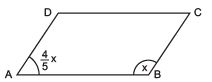∴ ∠ A= (4/5)x
Since, the adjacent angles of a parallelogram are supplementary.
∴ ∠ A + ∠ B = 180°
⇒ (4/5)x + x = 180°
⇒ 4x + 5x = 180° x 5
⇒ 9x = 180° x 5
⇒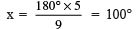∴ ∠ B = 100°
Since ∠ B= ∠ D            [Opposite angles of parallelogram]
∴ ∠ D = 100°
Now, ∠ A= (4/5)x =(4/5) x 100° = 80°
Also ∠ A= ∠ C             [Opposite angles of parallelogram]
∴ ∠ C = 80°
The required measures of the angles of the parallelogram are:  ∠ A = 80°, ∠ B = 100° ∠ C = 80° and ∠ D = 100°

Question 5. Find the measure of each angle of a parallelogram, if one of its angles is 15° less than twice the smallest angle.
Solution:
Let the smallest angle = x
Since, the other angle = (2x ∠ 15°)
Thus, (2x ∠ 15°) + x = 180°             [∵ x and (2x ∠ 15°) are the adjacent angles of a parallelogram]
⇒ 2x ∠ 15° + x = 180°
⇒ 3x ∠ 15° = 180°
⇒ 3x = 180° + 15° = 195°
⇒  x= (1950/3)= 65°
∴ The smallest angle = 65°
∴ The other angle = 2x - 15° = 2(65°) - 15° = 130° -15° = 115°
Thus, the measures of all the angles of parallelogram are: 65°, 115°, 65° and 115°.

Question 6. The lengths of the diagonals of a rhombus are 24 cm and 18 cm respectively. Find the length of each side of the rhombus.
Solution
: Since the diagonals of a rhombus bisect each other at right angles.
∴ O is the mid-point of AC and BD
⇒ AO =(1/2)AC and DO =(1/2)BD
Also ∠ AOD = 90°.
Now, ΔAOD is a right triangle, in which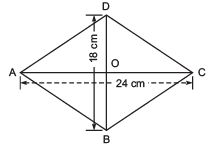AO = (1/2)AC =(1/2)(24 cm) = 12 cm
and DO = (1/2)BD =(1/2)(18 cm) = 9 cm
Since, AD2 = AO2 + DO2
⇒ AD2 = (12)2 + (9)2
= 144 + 81 = 225 = 152
⇒ AD = √(15)2 = 15
⇒ AD = AB = BC = CD = 15 cm (each)
Thus, the length of each side of the rhombus = 15 cm.

Question 7. One angle of a quadrilateral is of 108° and the remaining three angles are equal. Find each of the three equal angles.
Solution:
∴ ∠A + ∠B + ∠C + ∠D = 360°
⇒ 108° + [∠B + ∠C + ∠D] = 360°
⇒ [∠B + ∠C + ∠D]
= 360° - 108° = 252°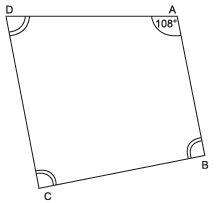Since,
∠D = ∠B = ∠C
∴ ∠B + ∠C + ∠D = 252°
⇒ ∠B + ∠B + ∠B = 252°
⇒  3∠B = 252°
⇒ ∠B = (2520/3) = 84°
∴ ∠B = ∠C = ∠D = 84°
Thus, the measure of each of the remaining angles is 84°.

Question 8. In the figure, AX and CY are respectively the bisectors of opposite angles A and C of a parallelogram ABCD. Show that AX || CY
Solution.
∵ ABCD is a ||gm
∴ Its opposite angles are equal.
⇒ ∠A = ∠C
⇒ (1/2)∠A =(1/2) ∠C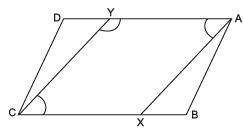i.e., ∠YAX = ∠YCX             ...(1)
Again DA || BC ⇒ YA || CX                [opposite sides of ||gm]
Also ∠AYC + ∠YCX = 180°            ...(2)
⇒ ∠AYC + ∠YAX = 180            [From (1) and (2)]
⇒ AX || CY              [As interior angles on the same side of the transversal are supplementary]

Question 9. E and F are respectively the mid points of the non-parallel sides AD and BC of a trapezium ABCD. Prove that: EF || AB and  EF = (1/2)(AB + CD)
Solution.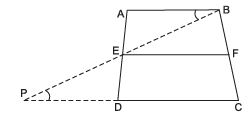Let us join BE and extend it meet CD produced at P.
In ΔAEB and ΔDEP, we get AB || PC and BP is a transversal,
∴ ∠ABE = ∠EPD             [Alternate angles]
AE = ED            [∵ E is midpoint of AB]
∠AEB = ∠PED             [Vertically opp. angles]
⇒ ΔAEB ≌ ΔDEP
⇒ BE = PE and AB = DP [SAS]
⇒ BE = PE and AB = DP
Now, in ΔEPC, E is a mid point of BP and F is mid point of BC
∴ EF || PC and EF =(1/2)PC            [Mid point theorem]
i.e., EF || AB and EF = (1/2) (PD + DC)
= (1/2) (AB + DC)
Thus, EF || AB and EF = (1/2) (AB + DC)

The document Short Answer Type Questions: Quadrilaterals - Notes | Study Mathematics (Maths) Class 9 - Class 9 is a part of the Class 9 Course Mathematics (Maths) Class 9.
All you need of Class 9 at this link: Class 9

## Mathematics (Maths) Class 9

88 videos|397 docs|109 tests
 Use Code STAYHOME200 and get INR 200 additional OFF

## Mathematics (Maths) Class 9

88 videos|397 docs|109 tests

Track your progress, build streaks, highlight & save important lessons and more!

,

,

,

,

,

,

,

,

,

,

,

,

,

,

,

,

,

,

,

,

,

;